# Free Vibration of a Spring Mass System

1.6 Experimentation

This experiment is for the free vibration analysis of a spring-mass system without any external damper. It involves a spring, a mass, a sensor, an acquisition system and a computer with a signal processing software as shown in Fig.1.4.

Accelerometer is used as a sensor to sense the motion of vibration and transfers the data to the computer through a data acquisition system. Data acquisition system takes vibration signal from the accelerometer and encodes it into digital form. Computer acts as a data storage and analysis system, and it takes encoded data from data acquisition system and processes the data using a signal processing software.

Experiments were done by taking two springs and two masses. So, total four experiments were done by choosing one spring and one mass at a time. Any two masses can be distinguished by their weights. Similarly, springs can be distinguished by their well known property called stiffness.

1.6.1 Spring Stiffness

a) Theoretical Calculation:

The stiffness of a spring is,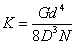(1.16)

where G is the Torsion Modulus,

d is the wire diameter,

D is the Mean coil diameter and D=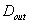-d; and

N is the number of active coils.

Table 1: Properties of different springs used in the experiment

 Spring Torsion modulus (G) in M Psi Wire diameter (d) in mm Outer coil diameter () mm Number of active coils A 10 0.90 7.983 62 B 10 0.90 6.46 81

###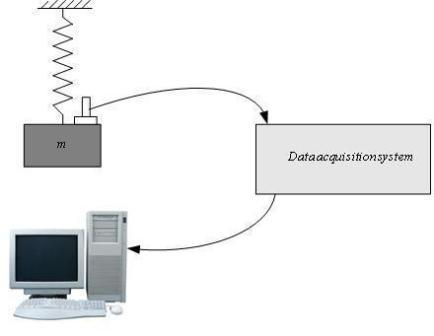Fig.1.4: Experimental Setup for the free vibration of a Spring-Mass System

###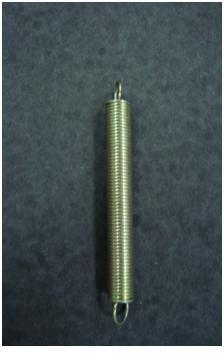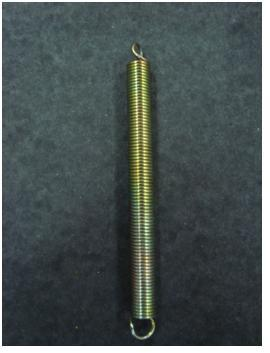Fig.1.5: (a) Spring A and (b) Spring B

b) Experimental Calculation:

The slope of the Load-Displacement plot of a system indicates its Stiffness.

###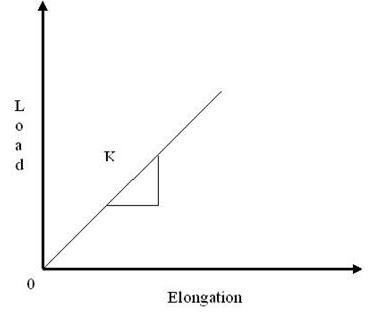1.6.2 Corrective Mass

Let us consider a mass less spring with stiffness k and has an effective mass, m eff , at the free end. Hence, the natural frequency of the spring-mass system without considering mass of accelerometer and mass of stand can be written as,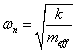(1.17)

Considering the mass of accelerometer and the mass of stand used to hang weights into account, the effective mass would be,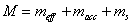(1.18)

Hence, the Natural frequency of the system considering the corrective mass will be,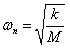(1.19)

1.6.3 Sample Calculations

Calculate the Natural Frequency of a spring-mass system with spring ‘A’ and a weight of 5N.

Solution:

Stiffness of spring ‘A’ can be obtained by using the data provided in Table 1, using Eq.(1.16)= 256.7 N/m

Using Eq. (1.17), corrective mass, M = (5/9.81) + 0.0182 + 0.1012 = 0.629 Kg.

Hence, the Natural Frequency of the system is,= 20.2 rad/sec.

1.6.4 Experimental Setup

The experimental setup of a spring-mass system contains a spring which is fixed to the support at one end by using a clamper and free at the other end as shown in Fig. 1.7. At the free end a stand is attached in order to place weights. Care must be taken while giving displacements to the mass in order to have motion only along the axis of the spring.

###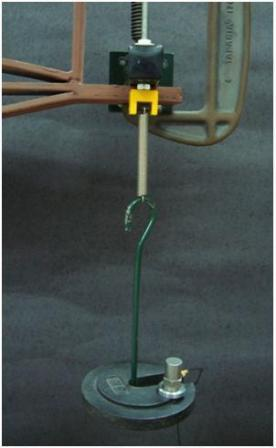Fig. 1.7: Experimental setup of a spring-mass system

###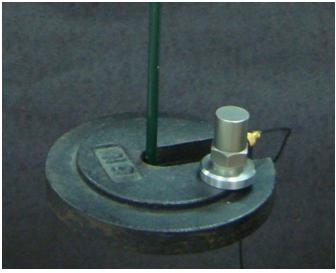Fig. 1.8: Accelerometer

### An accelerometer (Fig.1.8) is a time-dependent vibration measuring device. It is a transducer, which converts the acceleration of vibration into equivalent voltage signal, and sends it to data acquisition system. It is a contacting type of transducer.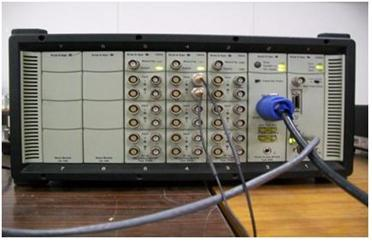Fig.1.9: Data acquisition system

### PULSE: Vibration measurement software shown in Fig. 1.10 uses the calibrated data from the data acquisition system for plotting the response.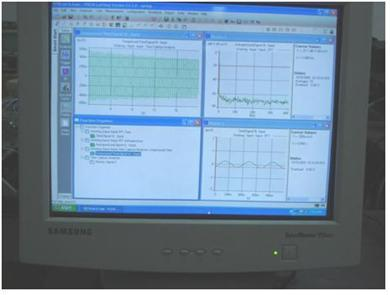Fig. 1.10: Time response from PULSE software